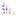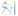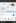1 Aug 2016, Samuel Hinton

# ChainConsumer

### A library I wrote to consumer chains to get parameter summaries and contours. I wanted to keep this project small, but after promoting it to the Dark Energy Survey, a got many requests for extensions. So now it can do many things, from LaTeX tables, convergence statistics, and so much more.

I was working with some chains one day and wanted to plot different cosmologies against each other, and realised that they had different parameters. Unfortunately, this meant that the library I was using couldn’t handle the task, so I wrote my own!

If you want to know more and see plenty of examples see the online documentation here.

The API is fairly straightforward I hope, with a simple example shown below.

``````import numpy as np
from numpy.random import normal, multivariate_normal
from chainconsumer import ChainConsumer

if __name__ == "__main__":
np.random.seed(0)
cov = normal(size=(3, 3))
cov2 = normal(size=(4, 4))
data = multivariate_normal(normal(size=3), 0.5 * (cov + cov.T), size=100000)
data2 = multivariate_normal(normal(size=4), 0.5 * (cov2 + cov2.T), size=100000)

c = ChainConsumer()
fig = c.plot()
``````

And the above code gives the following contour:The largest use of the library is to create corner plots like this one, and so most of the customisation methods, such that you can get the plot below.Upon request by various users, ChainConsumer now has extra functionality:

• Plot walks to provide a visual check for convergence
• Run converge diagnostics - the Gelman-Rubin statistic and Geweke diagnostic.
• Get parameter correlations and covariance - as a matrix or as a LaTeX table.
• Get parameter summaries, either as a python object or a LaTeX table.
• Compare models use the AIC, BIC and DIC tests.

Most of this is detailed in the glorious post I made but have yet had a chance to use!